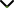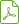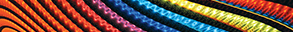# Analytical and Numerical Methods for Solving Partial Differential Equations and Integral Equations Arising in Physical Models 2014

Status
Published

Guest Editors

1National Institute of Technology, Rourkela, India

2National Institute of Technical Teachers’ Training and Research, Kolkata, India

3University Putra Malaysia (UPM), Selangor, Malaysia

4Southern Illinois University, Carbondale, USA

5Zhejiang University, Hangzhou, China

# Analytical and Numerical Methods for Solving Partial Differential Equations and Integral Equations Arising in Physical Models 2014

## DescriptionPartial differential equations (PDEs) have become a useful tool for describing the natural phenomena of science and engineering models. Nowadays, most of the phenomena that arise in mathematical physics and engineering fields can be described by PDEs. Many engineering applications are simulated mathematically as PDEs with initial and boundary conditions. Most physical phenomena of fluid dynamics, quantum mechanics, electricity, and many other models are controlled within their domain of validity by PDEs. Therefore, it becomes increasingly important to be familiar with all traditional and recently developed methods for solving PDEs and the implementations of these methods.

For many years the subject of functional equations has held a prominent place in the attention of mathematicians. In more recent years this attention has been directed to a particular kind of functional equation, an integral equation, wherein the unknown function occurs under the integral sign. Such equations occur widely in diverse areas of applied mathematics and physics. They offer a powerful technique for solving a variety of practical problems. One obvious reason for using the integral equation rather than differential equations is that all of the conditions specifying the initial value problems or boundary value problems for a differential equation can often be condensed into a single integral equation. Whether one is looking for an exact solution to a given problem or having to settle for an approximation to it, an integral equation formulation can often provide a useful way forward. For this reason integral equations have attracted attention for most of the last century.

This special issue is intended to present recent trends and advances of analytical and numerical methods for the solutions of partial differential equations and integral equations arising in physical models.

Potential topics include, but are not limited to:

• Recent developments of partial differential equation models in the real physical systems
• Mathematical modeling of integral equations in physical systems
• New reliable analytical and numerical methods for the solution of partial differential and integral equations
• Advances and applications of partial derivatives and integral equations in mechanics, electricity, economics, finance, biology, control theory, nonlinear waves, and chaos systemsDownload Special IssueJournal metrics
See full report
Acceptance rate13%
Submission to final decision68 days
Acceptance to publication21 days
CiteScore1.500
Journal Citation Indicator-
Impact Factor-Author guidelinesEditorial boardDatabases and indexing

Article of the Year Award: Outstanding research contributions of 2021, as selected by our Chief Editors. Read the winning articles.